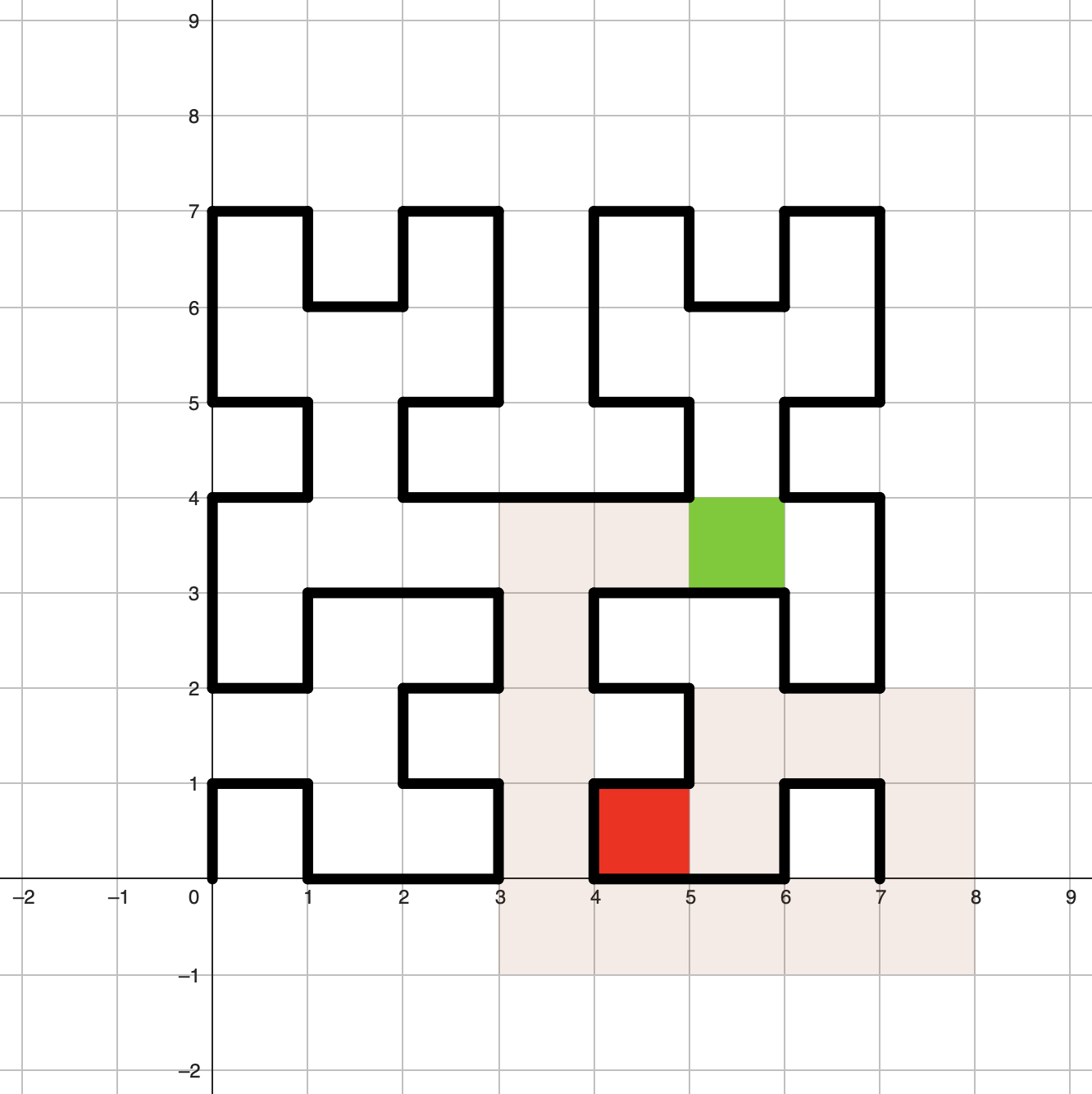시간 제한메모리 제한제출정답맞힌 사람정답 비율
1 초 1024 MB0000.000%

## 문제

David has elaborate hedge mazes in his garden. For each of his mazes of different sizes, he wonders how long it would take to walk between any pair of locations in and around that maze. A hedge maze of order $n\geq 1$ is constructed according to very specific instructions:

1. Start with $S=A$.
2. Repeat $n$ times:
1. Create a new string $T$ by replacing each $A$ in $S$ with $LBFRAFARFBL$ and each $B$ with $RAFLBFBLFAR$.
2. Set $S=T$.
3. Remove all $A$'s and all $B$'s from $S$.

The resulting string gives the instructions for constructing the hedge maze. In the unbounded plane start at coordinates $(0,0)$, facing towards $(1,0)$ and for every $F$ move forward one unit, for every $R$ turn right $90$ degrees, and for every $L$ turn left $90$ degrees.

After constructing the maze, identify position $(x,y)$ with the unit square cell bounded by coordinates $(x,y),(x+1,y),(x,y+1),(x+1,y+1)$. We can move directly between two positions $(x_1,y_1)$, $(x_2,y_2)$ if and only if either $|x_1-x_2|=1$ or $|y_1-y_2|=1$, but not both, and there is no hedge between these cells. The distance between these two positions is $1$.

Given a number $n$ and a pair of positions, find the shortest distance between these positions.

## 입력

Input starts with a positive integer $k$ $(1\leq k\leq 100)$, denoting the number of test cases. Each of the next $k$ lines consists of $5$ integers $n$, $x_1$, $y_1$, $x_2$, $y_2$, satisfying $1\leq n\leq 50$, and $-2^{52}\leq x_1,y_1,x_2,y_2\leq 2^{52}$. There is no interaction between the test cases. Each specifies a single hedge maze situated alone in the unbounded plane.

## 출력

Output $k$ lines with one integer on each line, corresponding to the shortest distance between the two positions in each of the $k$ test cases.

## 예제 입력 1

2
3 5 3 4 0
2 4 3 0 2


## 예제 출력 1

16
11


## 힌트The shortest path from the first case in the sample input.